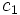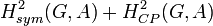# Second cohomology group for trivial group action of E8 on Z2

This article gives information about the second cohomology group for trivial group action (i.e., the second cohomology group with trivial action) of the group elementary abelian group:E8 on cyclic group:Z2. The elements of this classify the group extensions with cyclic group:Z2 in the center and elementary abelian group:E8 the corresponding quotient group. Specifically, these are precisely the central extensions with the given base group and acting group.
The value of this cohomology group is elementary abelian group:E64.
Get more specific information about elementary abelian group:E8 |Get more specific information about cyclic group:Z2|View other constructions whose value is elementary abelian group:E64

## Description of the group

We consider here the second cohomology group for trivial group action of elementary abelian group:E8 on the cyclic group:Z2, i.e.,$\! H^2(G,A)$

where$G \cong E_8 \cong \mathbb{Z}_2 \times \mathbb{Z}_2 \times \mathbb{Z}_2$ and$A \cong \mathbb{Z}_2$.

The cohomology group is isomorphic to elementary abelian group:E64.

## Elements

We list here the elements, grouped by similarity under the action of the automorphism groups on both sides.

Cohomology class type Number of cohomology classes Trace Representative 2-cocycle Corresponding group extension Second part of GAP ID (order is 16)
trivial 1 0$0$ everywhere elementary abelian group:E16 14
symmetric and nontrivial 7 4 PLACEHOLDER FOR INFORMATION TO BE FILLED IN: [SHOW MORE] direct product of Z4 and V4 10
one of the non-symmetric ones 28 4 PLACEHOLDER FOR INFORMATION TO BE FILLED IN: [SHOW MORE] central product of D8 and Z4 13
one of the non-symmetric ones 21 2 PLACEHOLDER FOR INFORMATION TO BE FILLED IN: [SHOW MORE] direct product of D8 and Z2 11
one of the non-symmetric ones 7 6 PLACEHOLDER FOR INFORMATION TO BE FILLED IN: [SHOW MORE] direct product of Q8 and Z2 12

Here, the trace of a cohomology class is defined as the trace of any normalized 2-cocycle representing that cohomology class, written as a$|G| \times |G|$ matrix. This is well defined because all the normalized 2-coboundaries are IIP, i.e., they have zero trace. Further information: normalized 2-coboundary for trivial group action of elementary abelian 2-group of Z2 is IIP

This trace can also be interpreted as the number of cosets of$A$ in the big extension group that have elements of order$4$.

Note that under the group operation of$H^2(G,A)$, the trace map gets added up to parity. In other words, for cohomology classes$c_1$ and$c_2$, the trace of$c_1 + c_2$ has the same parity as the sum of the traces of$c_1$ and$c_2$.

## Group actions

### Under the action of the automorphism group of the acting group

The acting group$G$, which is isomorphic to elementary abelian group:E8, has a huge automorphism group, general linear group:GL(3,2) of order$168$. Each cohomology class type in the table above is one orbit. In particular, the trivial group extension is the only fixed point, there are two orbits of size$7$, and there is one orbit each of size$21$ and$28$.

## Subgroups of interest

Subgroup Value Corresponding group extensions for subgroup GAP IDs second part Group extension groupings for each coset GAP IDs second part
IIP subgroup of second cohomology group for trivial group action trivial group elementary abelian group:E16 14 elementary abelian group:E16 (1 copy), direct product of Z4 and V4 (7 copies), central product of D8 and Z4 (28 copies), direct product of D8 and Z2 (7 copies), direct product of Q8 and Z2 (21 copies) 14 (1 copy), 10 (7 copies), 14 (28 copies), 11 (21 copies), 12 (7 copies)
cyclicity-preserving subgroup of second cohomology group for trivial group action trivial group elementary abelian group:E16 14 elementary abelian group:E16 (1 copy), direct product of Z4 and V4 (7 copies), central product of D8 and Z4 (28 copies), direct product of D8 and Z2 (7 copies), direct product of Q8 and Z2 (21 copies) 14 (1 copy), 10 (7 copies), 14 (28 copies), 11 (21 copies), 12 (7 copies)
subgroup generated by images of symmetric 2-cocycles (corresponds to abelian group extensions) elementary abelian group:E8 elementary abelian group:E16, direct product of Z4 and V4 (7 times) 14 (1 copy), 10 (7 copies) (elementary abelian group:E16, direct product of Z4 and V4 (7 times)) (1 copy), (direct product of Q8 and Z2, direct product of D8 and Z2 (3 times), central product of D8 and Z4 (4 times)) (7 copies) (14, 10 (7 times)) (1 copy), (12, 11 (3 times), 13 (4 times)) (7 copies)

## Direct sum decomposition

For background information, see formula for second cohomology group for trivial group action of abelian group in terms of Schur multiplier and abelianization

### General background

We know from the general theory that there is a natural short exact sequence:$0 \to \operatorname{Ext}^1_{\mathbb{Z}}(G,A) \to H^2(G;A) \stackrel{\operatorname{Skew}}{\to} \operatorname{Hom}(\bigwedge^2G,A) \to 0$

where the image of$\operatorname{Ext}^1$ is$H^2_{sym}(G;A)$, i.e., the group of cohomology classes represented by symmetric 2-cocycles. We also know, again from the general theory, that the short exact sequence above splits, i.e.,$H^2_{sym}(G;A)$ has a complement inside$H^2$. However, there need not in general be a natural or even an automorphism-invariant choice of splitting.

### In this case

For this choice of$G$ and$A$, the subgroup$H^2_{CP}(G;A)$ of cyclicity-preserving cohomology classes is trivial, hence the sum$H^2_{sym}(G;A) + H^2_{CP}(G;A)$ is$H^2_{sym}(G;A)$ and is not the whole group$H^2(G;A)$. Thus,$H^2_{CP}(G;A)$ is not the desired complement. For this choice of$G$ and$A$, the subgroup$H^2_{CP}(G,A)$ of cyclicity-preserving cohomology classes is trivial, hence the sum$H^2_{sym}(G,A) + H^2_{CP}(G,A)$ is$H^2_{sym}(G,A)$ and is not the whole group$H^2(G,A)$. (The same holds true if we replace$H^2_{CP}(G,A)$ with$H^2_{IIP}(G,A)$).

In fact, there is no subgroup complement to$H^2_{sym}(G,A)$ in$H^2(G,A)$ that is invariant under the action of$\operatorname{Aut}(G) \times \operatorname{Aut}(A)$.

## GAP implementation

### Construction of the cohomology group and extensions

The cohomology group and the corresponding extensions can be constucted using the GAP functions ElementaryAbelianGroup, TwoCohomology, TrivialGModule, GF.

gap> G := ElementaryAbelianGroup(8);;
gap> A := TrivialGModule(G,GF(2));;
gap> T := TwoCohomology(G,A);
rec( group := <pc group of size 8 with 3 generators>,
module := rec( field := GF(2), isMTXModule := true, dimension := 1,
generators := [ <an immutable 1x1 matrix over GF2>,
<an immutable 1x1 matrix over GF2>,
<an immutable 1x1 matrix over GF2> ] ),
collector := rec( relators :=
[ [ 0 ], [ [ 2, 1 ], 0 ], [ [ 3, 1 ], [ 3, 1 ], 0 ] ],
orders := [ 2, 2, 2 ], wstack := [ [ 1, 1 ], [ 2, 1 ] ],
estack := [  ], pstack := [ 3, 3 ], cstack := [ 1, 1 ],
mstack := [ 0, 0 ], list := [ 0, 0, 0 ],
module := [ <an immutable 1x1 matrix over GF2>,
<an immutable 1x1 matrix over GF2>,
<an immutable 1x1 matrix over GF2> ],
mone := <an immutable 1x1 matrix over GF2>,
mzero := <an immutable 1x1 matrix over GF2>, avoid := [  ],
unavoidable := [ 1, 2, 3, 4, 5, 6 ] ),
cohom := <linear mapping by matrix, <vector space of dimension 6 over GF(
2)> -> ( GF(2)^6 )>,
presentation := rec( group := <free group on the generators [ f1, f2, f3 ]>,
relators := [ f1^2, f1^-1*f2*f1*f2^-1, f2^2, f1^-1*f3*f1*f3^-1,
f2^-1*f3*f2*f3^-1, f3^2 ] ) )

### Construction of extensions

The extensions can be constructed using the additional command Extensions.

gap> G := ElementaryAbelianGroup(8);;
gap> A := TrivialGModule(G,GF(2));;
gap> L := Extensions(G,A);;
gap> List(L,IdGroup);
[ [ 16, 14 ], [ 16, 10 ], [ 16, 11 ], [ 16, 11 ], [ 16, 11 ], [ 16, 11 ],
[ 16, 11 ], [ 16, 11 ], [ 16, 10 ], [ 16, 10 ], [ 16, 11 ], [ 16, 12 ],
[ 16, 13 ], [ 16, 13 ], [ 16, 13 ], [ 16, 13 ], [ 16, 11 ], [ 16, 13 ],
[ 16, 11 ], [ 16, 13 ], [ 16, 11 ], [ 16, 13 ], [ 16, 13 ], [ 16, 11 ],
[ 16, 11 ], [ 16, 13 ], [ 16, 11 ], [ 16, 13 ], [ 16, 13 ], [ 16, 11 ],
[ 16, 11 ], [ 16, 13 ], [ 16, 10 ], [ 16, 10 ], [ 16, 13 ], [ 16, 13 ],
[ 16, 11 ], [ 16, 12 ], [ 16, 13 ], [ 16, 13 ], [ 16, 10 ], [ 16, 10 ],
[ 16, 13 ], [ 16, 13 ], [ 16, 13 ], [ 16, 13 ], [ 16, 11 ], [ 16, 12 ],
[ 16, 11 ], [ 16, 13 ], [ 16, 13 ], [ 16, 11 ], [ 16, 11 ], [ 16, 13 ],
[ 16, 11 ], [ 16, 13 ], [ 16, 12 ], [ 16, 13 ], [ 16, 13 ], [ 16, 12 ],
[ 16, 13 ], [ 16, 12 ], [ 16, 13 ], [ 16, 12 ] ]

### Under the action of the various automorphism groups

This uses additionally the GAP functions AutomorphismGroup, DirectProduct, CompatiblePairs, and ExtensionRepresentatives.

gap> G := ElementaryAbelianGroup(8);;
gap> A := TrivialGModule(G,GF(2));;
gap> A1 := AutomorphismGroup(G);;
gap> A2 := GL(1,2);;
gap> D := DirectProduct(A1,A2);;
gap> P := CompatiblePairs(G,A,D);;
gap> M := ExtensionRepresentatives(G,A,P);;
gap> List(M,IdGroup);
[ [ 16, 14 ], [ 16, 10 ], [ 16, 11 ], [ 16, 12 ], [ 16, 13 ] ]